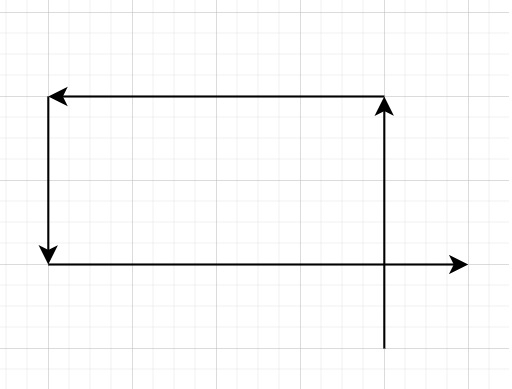# Self Crossing in C++

Suppose we have an array x of n numbers. We start at point (0,0) and moves x units to the north direction, then x units to the west direction, x units to the south direction , x units to the east direction and so on. In other words, after each move our direction changes counter-clockwise. We have to devise an one-pass algorithm with O(1) extra space to determine whether our path crosses itself, or not.

So if the array is like − [3,4,2,5]To solve this, we will follow these steps −

• insert 0 at the index 4 of x

• n := size of x, i := 4

• for not initializing anything when i < n and x[i] > x[i - 2], increase i by 1 do −

• Do nothing

• if i is same as n, then, return false

• if x[i] >= x[i - 2] - x[i - 4], then,

• x[i - 1] = x[i - 1] - x[i - 3]

• for increase i by 1, when i < n and x[i] < x[i - 2], increase i by 1 do −

• Do nothing

• return true when i is not same as n

## Example

Let us see the following implementation to get better understanding −

Live Demo

#include <bits/stdc++.h>
using namespace std;
class Solution {
public:
bool isSelfCrossing(vector<int>& x) {
x.insert(x.begin(), 4, 0);
int n = x.size();
int i = 4;
for(; i < n && x[i] > x[ i - 2];i++);
if(i == n) return false;
if (x[i] >= x[i - 2] - x[i - 4]){
x[i - 1] -= x[i - 3];
}
for (i++; i < n && x[i] < x[i - 2]; i++);
return i != n;
}
};
main(){
Solution ob;
vector<int> v = {3,4,2,5};
cout << (ob.isSelfCrossing(v));
}

## Input

{3,4,2,5}

Output

1